# Team:Edinburgh/modelling(reallifemodelling)

Our WIKI is best viewed with (click title):

* Internet Explorer 8
* Firefox 3

Modelling - Real Life Modelling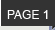Personal note

One of the exciting things about iGEM, is that I got the oppertunity to widen my knowledge, not just in Engineering, but also in Biology and Informatics. This project has had such an impact on me that I will actually continue my masters project on it! I will be characterising the TNT receptor that we require for our system.

Rachel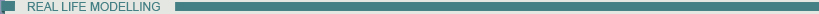Since our system designed to detect landmines it was thought that we should include a visual representation of how the system would operate in real life. It is known that TNT leaks out of the landmine into the soil and we predict that the bacteria will move toward the TNT due to the chemotaxis response of the ribose binding protein which has previously computationally designed TNT receptor1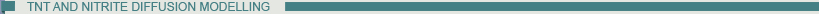TNT and Nitrite diffusion has been modelled using a finite difference method which approximates the 2 dimensional time dependent diffusion equation below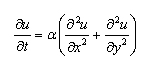where α is the diffusion coefficient, to a numerical solution which can be solved . By splitting the area into a grid M2 by N with a space step of h=1/M (in both the x and y directions) and time step size of k=T/N. This gives a grid of the form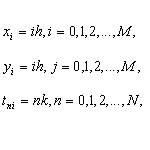which was set up as a matrix in the program.

An initial concentration of TNT in the field was then set to 107800µg/cm3 and the diffusion of TNT out from this site was calculated from the following equation,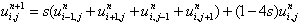and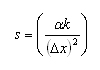The diffusion coefficient for TNT is 1.18 *10-6 cm2 s-1 4 and the diffusion of Nitrite is 1.9*10-5 cm2 s-1 .

This system was coded in both MATLAB and JAVA. MATLAB was used to produce graphs and JAVA was used for the chemotaxis modelling which is described below.

The TNT diffusion graph after a year is shown below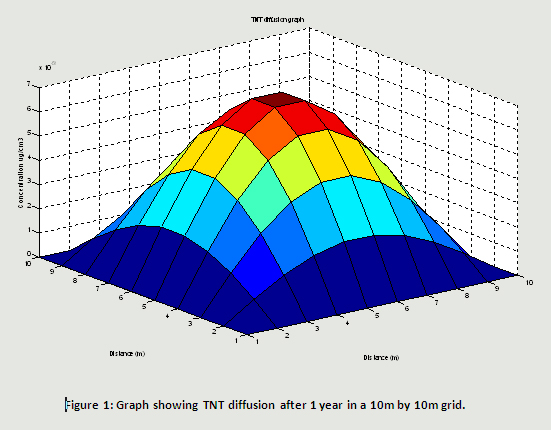Figure 1 shows the diffusion of TNT over a small area, only 10m by 10m, so that the uniform distribution of the TNT concentration can be shown. However a better representation of what would happen in the field can be shown when the size of the grid is much larger, 100m by 100m as shown below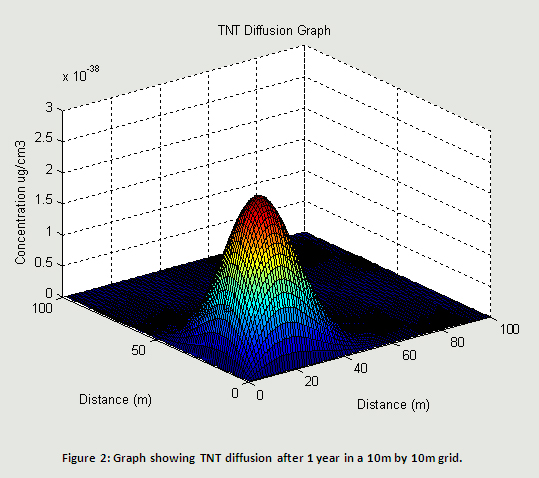It is clear from Figure 2 that TNT is only concentrated around the landmines location rather than across the whole field and hence the bacteria could be used to determine the approximate location of the landmine due to the light emit on detection of the TNT. The bacteria also produces enzymes, nitroreducases, which breaks down TNT into various products including Nitrites. Our system is designed to emit light when these Nitrites are detected. For the model it has been assumed that a very low conversion of TNT to Nitrites will be achieved. The diffusion graph for Nitrite is very similar to that for TNT.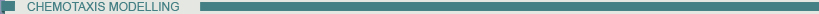As previously stated, it is expected that the bacteria will move toward the landmine due to chemotaxis of the TNT receptor. This was incorporated into the model by assuming the bacteria will move toward the highest concentration of TNT, essentially swimming up the concentration gradient. This was programmed by incorporating a 3rd matrix where the grid matches up with both the TNT and the Nitrite diffusion graphs. The bacteria is then placed in the matrix using a random number generator to get its grid location and this location is set to 1 while the rest of the grid is set to zero.

The model then calculates the concentration of TNT at its grid position and its 4 surrounding grid positions. It then stores the current concentration as the maximum concentration and then compares each of the surrounding locations concentrations to this maximum. If any of the concentrations are greater than the current locations concentration then it set this concentration as the maximum and will then compare subsequent concentrations to this new maximum. It also indexes the location of the maximum concentration which is used to determine where the bacteria should move to. The moving of the bacteria is done by a series of IF statements as shown below

if (index==1){
Bact[xbact][ybact]=1;
}

if (index==2){
Bact[xbact-1][ybact]=1;
xbact=xbact-1;
}

if (index==3){
Bact[xbact+1][ybact]=1;
xbact=xbact+1;
}

if (index==4){
Bact[xbact][ybact-1]=1;
ybact=ybact-1;
}

if (index==5){
Bact[xbact][ybact+1]=1;
ybact=ybact+1;
}

Depending on the value of the index, depend on where the bacteria moves to by setting the new location in the grid for the bacteria to 1 and by reseting the location of the bacteria.

The random motion of chemotaxis has not been included in the model as over the distances that system operates it was thought that this would be unimportant and in general it would travel in a straight line.

This model is shown in an applet where the background is coloured green to represent a field, the landmine is coloured black and the bacteria is coloured yellow.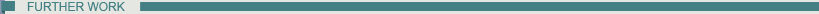The model could be developed further by introducing more bacteria which would all move towards the highest concentration of TNT. Further to this the model could incorporate the concentration of Nitrite and dependant on the concentration of TNT and Nitrite the bacteria could be shown to represent the colour that would experienced in field, ie there would be a yellow and blue circle where the bacteria are. Further to this, the model could be linked with the gene expression modelling so that the colour of the light emitted could be dependent on the level of each protein expressed at each location.

It has also been suggested that the model could be made into a game, similar to minesweeper, where you use the movement of the bacteria to determine where the landmine is located.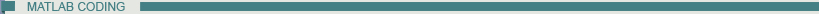display ('***********************************************************')
%Finite difference model for the diffusion of TNT through soil

xsize=100;
ysize=100;
MaxSteps=8760;
TimeStep=0.01;
SpaceStep=1;
diffusivityC=1.18*(10-6)*3600/100/100;
%calculates s and s2 for TNT based on the diffusion coefficient of TNT
sC=(diffusivityC*TimeStep/SpaceStep/SpaceStep);
s2C=(1-4*sC);
diffusivityN=1.18*(10-6)*3600/100/100;
%Calculates s and s2 for Nitrites based on the diffusion coefficient of
%Nitrites
sN=(diffusivityN*TimeStep/SpaceStep/SpaceStep);
s2N=(1-4*sC);

% Sets up 2 matricies for the calculation
C=zeros (xsize,ysize);
CNew = zeros (xsize,ysize);
N= zeros (xsize,ysize);
NNew= zeros (xsize,ysize);

%Set up the concentration and position of the landmine
MineX=5;
MineY=5;
C(MineX,MineY)=107800;
N (MineX,MineY)=1;

React=0.99;
Produced=1/React;

for k=1:MaxSteps,
for i=2:xsize-1,
for j=2 :ysize-1,
%Calculates concentrations due to finite difference equation
CNew(i,j)= React*(sC*(C((i-1),j)+ C((i+1),j)+C(i,(j-1))+C(i,(j+1)))+s2C*C(i,j));
NNew(i,j)=Produced*(sN*(N((i-1),j)+ N((i+1),j)+N(i,(j-1))+N(i,(j+1)))+s2N*N(i,j));

end
end

C=CNew;
N=NNew;
display (C)
display(N)
>br /> surfc (C)

refreshdata

end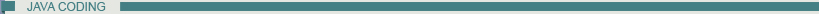import java.applet.*;
import java.awt.*;
import java.awt.event.*;
import java.util.Random;

import javax.swing.JOptionPane;

public class ChemotaxisModel extends Applet implements Runnable {

/**
*
*/
private static final long serialVersionUID = 1L;

int h,j,k1,score,x1,y1,xmove,ymove,cloud,xsize1,ysize1,mx,my,cx,cy, c1;
Graphics offscreen;
Image workspace;
Color vdkgreen;
int MineX, MineY;
String UserMineX, UserMineY;{

UserMineX=JOptionPane.showInputDialog( "Enter x-location for the landmine" );
UserMineY=JOptionPane.showInputDialog("Enter y-location for the landmine");

MineX=Integer.parseInt(UserMineX);
MineY=Integer.parseInt(UserMineY); }

public ChemotaxisModel () {}

public void init() {
xsize1=getSize().width;
ysize1=getSize().height;
workspace=createImage(xsize1,ysize1);
offscreen=workspace.getGraphics();
x1=100;y1=100;xmove=0;ymove=0;

vdkgreen=new Color(0,102,0);

}

public void start (){
if (t==null){
t.start();

}
}
public void run () {}

public void stop (){
if (t != null){
t.stop ();
t=null;
}
}

public void paint (Graphics screen){

offscreen.setColor(vdkgreen);
offscreen.fillRect(0,0,xsize1,ysize1);

drawBacteria (offscreen);
drawLandmine (offscreen);
screen.drawImage(workspace,0,0,this);
}

public void update(Graphics screen){
paint(screen);
}

void drawBacteria (Graphics g){

int xsize, ysize, c1, c2, c3, indexx,indexy,xbact,ybact;
long c4,c5,c6,c7,c8;
double C[][],N[][],Bact[][],BactSurr[];
double NewC[][],NewN[][], NewBact[][];
double TimeStep, SpaceStep, Alpha,s, s2, k,x,maxC,max,index;

double TNTLoss,N2Prod,TotalTNT, TotalNitrite;

int MaxSteps=1000;

xsize=10;
ysize=10;
C=new double[xsize][ysize];
NewC=new double[xsize][ysize];
N=new double [xsize][ysize];
NewN=new double [xsize][ysize];
Bact=new double [xsize][ysize];
NewBact=new double [xsize][ysize];
BactSurr=new double;
TimeStep=0.01;
SpaceStep=1;
Alpha=0.2;
s=(Alpha*TimeStep/SpaceStep/SpaceStep);
s2=(1-4*s);

for (c1=0; c1 for (c2=0; c2 C[c1][c2]=0;
N[c1][c2]=0;
Bact [c1][c2]=0;
}
}
C[MineX][MineY]=1000;
N[MineX][MineY]=10;
k=0.06;
TNTLoss=1;
N2Prod=1/TNTLoss;
TotalTNT=0;
TotalNitrite=0;
x=0;
index=0;
indexx=0;
indexy=0;
max=0;

max=0;
Random generator = new Random();
Random generator2 = new Random();
xbact = generator.nextInt( xsize-5 )+2;
ybact = generator2.nextInt( ysize-5 )+2;
Bact[xbact][ybact]=1;
for (c3=0;c3 for (c1=1; c1< (xsize-1); c1++) {
for (c2=1; c2< (ysize-1); c2++) {
NewC[c1][c2]=TNTLoss*(s*(C[c1-1][c2]+C[c1+1][c2]+C[c1][c2-1]+C[c1][c2+1])+s2*C[c1][c2]);
NewN[c1][c2]=N2Prod*(s*(N[c1-1][c2]+N[c1+1][c2]+N[c1][c2-1]+N[c1][c2+1])+s2*N[c1][c2]);
TotalTNT +=(C[c1][c2]);
TotalNitrite +=N[c1][c2];
}
}

System.out.println("TNT");
for (c1=1; c1< (xsize-1); c1++) {
for (c2=1; c2< (ysize-1); c2++) {

c4=Math.round(C[c1][c2]);
System.out.print(c4);
System.out.print("-");
C[c1][c2]=NewC[c1][c2];
}
System.out.println(".");
}
c6=Math.round(TotalTNT);
System.out.println("*");
System.out.println(c6);
System.out.println("*");
TotalTNT=0;
}

for (c3=94;c3
System.out.println("Bacteria");
for (c1=1; c1< (xsize-1); c1++) {
for (c2=1; c2< (ysize-1); c2++) {

c8=Math.round(Bact[c1][c2]);
System.out.print(c8);
System.out.print("-");
Bact[c1][c2]=NewBact[c1][c2];
}
System.out.println(".");
}

BactSurr=C[xbact][ybact];
BactSurr=C[xbact-1][ybact];
BactSurr=C[xbact+1][ybact];
BactSurr=C[xbact][ybact-1];
BactSurr=C[xbact][ybact+1];

System.out.println(BactSurr);
System.out.println(BactSurr);
System.out.println(BactSurr);
System.out.println(BactSurr);
System.out.println(BactSurr);

max=0;
index=0;

for (c1=1; c1<6;c1++){
if (max max=BactSurr[c1];
index=c1;
System.out.println(index);
}
} System.out.println(index);

if (index==1){
Bact[xbact][ybact]=1;
}

if (index==2){
Bact[xbact-1][ybact]=1;
xbact=xbact-1;
}

if (index==3){
Bact[xbact+1][ybact]=1;
xbact=xbact+1;
}

if (index==4){
Bact[xbact][ybact-1]=1;
ybact=ybact-1;
}

if (index==5){
Bact[xbact][ybact+1]=1;
ybact=ybact+1;
}

g.setColor(Color.yellow);
g.fillOval(xbact*20, ybact*20, 5, 5);
}
}

void drawLandmine (Graphics g){
g.setColor (Color.black);
g.fillOval(MineX*20,MineY*20, 10,10);
}
}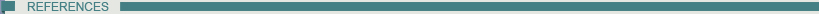1Looger, L, Dwyer, M, Smith, J, Hellinga, H, “Computational design of receptor and sensor proteins with novel functions”, Nature, vol. 423, pp185-190.

2Dehghan, M, “Fully explicit finite-difference methods for two-dimensional diffusion with an integral condition”, Nonliner Analysis, vol.48, p637-650, 2002.

3B. Baez et al, “Detection of chemical signatures from TNT buried in sand at various ambient conditions: phase II” Detection and Remediation Technologies for Mines and Minelike Targets XI, SPIE International Society Optical Engineering, Orlando, FL, USA (2006) pp. 62171M..

4Valsaraj, K.T et al, “Diffusive transport of 2, 4, 6-trinitrotoluene (TNT) from contaminated soil to overlying water”, Journal of Hazardous Materials, vol.59, p1-12, 1998.

5Schramm, A et al, “Structure and Function of a Nitrifying Biofilm as Determined by In Situ Hybridization and the Use of Microelectrodes”, Applied and Environmental Microbiology, Vol.62, No.12 p4641-4647, Dec. 1996.
Edinburgh University iGEM Team 2009